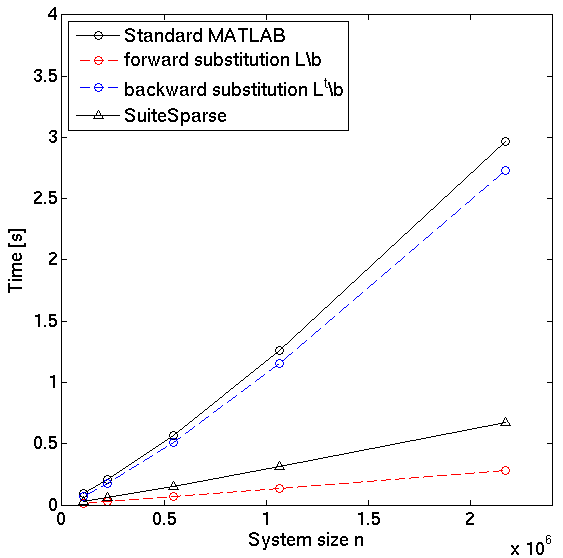# Forward and Backward substitution

Once the system matrix A has been factorized using the Cholesky factorization the original linear system of equations can be represented as

LLTxperm = bperm

Note that the x and b vectors are permuted using the fill-reducing reordering used. The permuted solution vector xperm is obtained using forward substitution

LTxperm = L-1bperm

followed by backward substitution

xperm = (LT)-1L-1bperm

Using MATLAB the above can be implemented as

Code Fragment 1:

`x(Free_ind(perm)) = L'\(L\b(Free_ind(perm)));`

where perm is the permutation vector and Free_ind is a vector holding the indices of unconstrained nodes (see the Boundary Conditions section).

Since the same number of floating point operations needs to be performed in the forward (L\) and backward (L’\) substitutions, in the ideal scenario each step should take the same amount of time. However, in the above implementation the factor L needs to be explicitly transposed in order to perform backward substitution. The additional work causes this implementation to be sub-optimal.

One option is to implement the above using the ‘/’ (or mrdivide MATLAB function). This avoids explicit computation of L’, i.e., only L is used in the MATLAB code:

Code Fragment 2:

`x(Free_ind(perm)) = (L\b(Free_ind(perm)))'/L;`

However, according to the documentation mrdivide performs the transposition of the matrix internaly:

/ Slash or right matrix divide.
A/B is the matrix division of B into A, which is roughly the
same as A*INV(B) , except it is computed in a different way.
More precisely, A/B = (B’\A’)’. See MLDIVIDE for details.

Effectively, both above implementations have the same performance.

The performance of backward substitution can be significantly improved with the help of the external library SuiteSparse. The function cs_ltsolve uses the lower-triangular factor L and performs the backward substitution without explicit matrix transposition

Code Fragment 3:

`x(Free_ind(perm)) = cs_ltsolve(L,cs_lsolve(L,b(Free_ind(perm))));`

As seen in Figure 1, this has a major impact on performance. Using SuiteSparse to perform both the forward and backward substitution improves the performance roughly four times over a native MATLAB implementation. The red curve shows the time needed to perform the native MATLAB forward substitution (L\) only. It takes roughly half the time needed by the SuiteSparse implementation to compute both substitution steps, which agrees with our expectations that the two stages should take the same time. The blue curve shows the time taken by the native MATLAB backward substitution (L’\). Clearly, the speedup of SuiteSparse comes from avoiding the explicit transposition of the factor.Performance of forward and backward substitution using native MATLAB and SuiteSparse implementations.